# Detect and Remove Loop in a Linked List

July 13, 2020 16219

Given a singly Linked List, detect if it contains a loop or not and if so, remove the loop.

Input: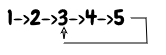Output: 1 → 2 → 3 → 4 → 5 → NULL

Input: 1→ 2→ 3→ NULL

Output: 1 → 2 → 3 → NULL

To remove a loop in a Linked List, we need to get the pointer of the last node and make it’s next pointer to NULL. But before removing a loop, we must first detect it and the same is discussed in the article Detect a loop in linked list using Floyd’s Cycle Finding algorithm. So now I hope you are familiar with the fast and slow pointer approach of Floyd’s algorithm to detect a loop in a Linked List. Taking this concept further, follow the below algorithm,

1. So, if there is a loop detected, then our ptr1 and ptr2 will be pointing to the same node inside a loop.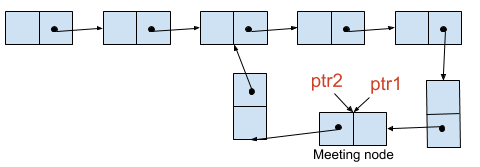2. Now assign head node of the Linked List to ptr2 and keep ptr1 at the same node (at the meeting point).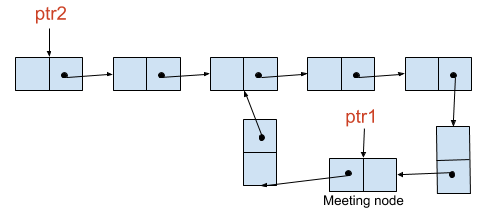3. Again move forward both the pointers from their respective positions but this time both at the same speed, that is one node at a time, until the next of ptr1 and ptr2 meet. And at this time, the next of both the pointers will meet at the start node of the loop and ptr2 will be pointing at the last node of the linked list.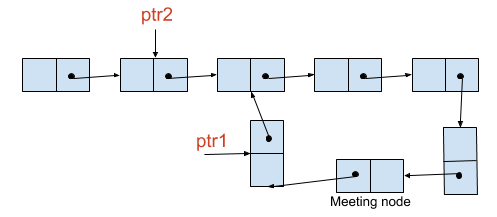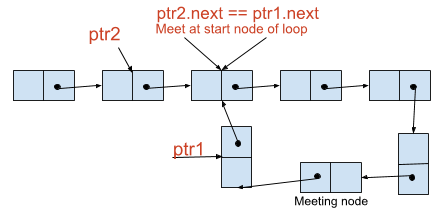4. Hence, assign Null to the next of ptr1 and stop the execution.

Find below the working of the above algorithm,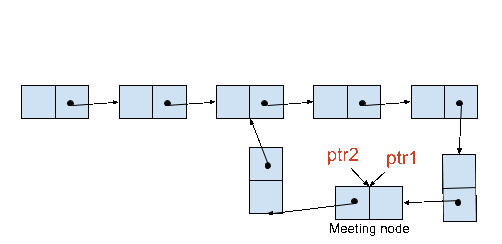The time complexity of Detect and Remove Loop in a Linked List using the above approach is $O(n)$.

## Why ptr1 and ptr2 will meet at the start node of the loop in the above algorithm?

Remember we left our pointers ptr1 and ptr2 at the position shown in the below diagram when we detected a loop in the Linked List. Taking the same configuration, first, let’s find out the distance traveled by ptr1 to reach the meeting point in terms of length of loop L and the total length of Linked List n.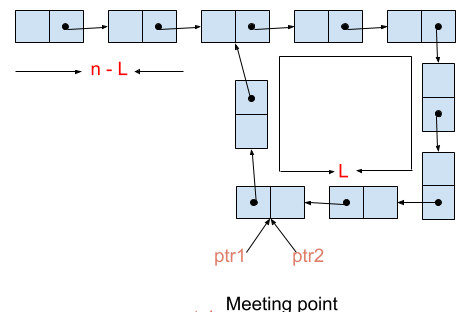From the below figure, we can easily conclude that when ptr1 initially reached the start node of the loop, it has covered (n-L) steps and ptr2 would have covered 2*(n-L) steps that is extra (n-L) steps inside the loop. So at this stage, the effective gap between ptr1 and ptr2 would be (n-L)%L which is equivalent to n%L. Now both the pointers will meet inside the loop when the gap becomes L. Therefore, ptr1 has to travel further (L - n%L) steps to reach the meeting point since the current gap is n%L. Hence at (L-n%L) steps from the start node of the loop, both the pointers will meet.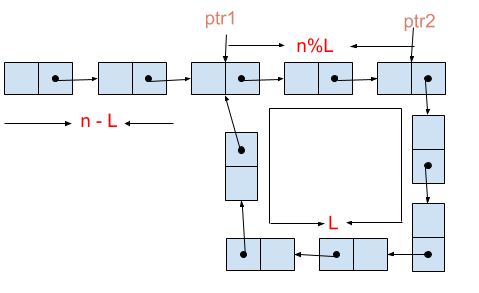Now we move ptr1 to the head node of the Linked List and keep ptr2 at the meeting point. ptr1 will take (n-L) steps to reach the start node of the loop and since we are moving ptr2 with the same speed as ptr1, that is one node at a time, it would also move (n-L) steps inside the loop. Therefore, at this stage, the current position of ptr2 w.r.t to start node of the loop would be (L -n%L + (n-L))%L which simplifies to (n-n%L)%L and that is equal to zero. Hence, ptr2 is also at the start node of the loop.

In our case, before moving ptr1 and ptr2 forward we are checking if the next of ptr1 and ptr2 is same because this way we can stop ptr2 at the last node of the loop and make its next NULL to remove the loop.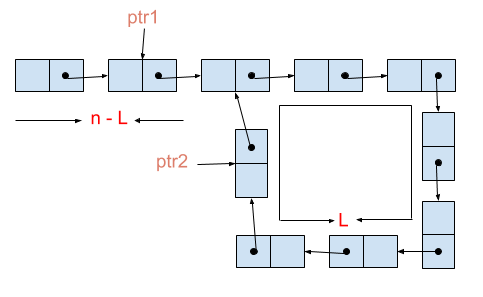Below is the implementation of the above algorithm,

• C/C++
• Python
• Java
#include <stdio.h>
#include <stdlib.h>

struct ListNode
{
int val;
struct ListNode *next;
};

{
// Initialize ptr1 and ptr2 to head node.
// Boolean to check if there a loop exists in the given Linked List.
int flag = 0;
// Traverse the list until ptr2 or ptr2.next is null.
while (ptr2 != NULL && ptr2->next != NULL)
{
// Move ptr1 forward by one node.
ptr1 = ptr1->next;
// Move ptr2 forward by two nodes.
ptr2 = ptr2->next->next;
// Check if ptr1 and ptr2 meet at some node, then there is a loop in the Linked List and So make flag true and break.
if (ptr1 == ptr2)
{
flag = 1;
break;
}
}
// When there is a loop in the Linked List.
if (flag)
{
// Loop until next of ptr1 and ptr2 are not equal.
while (ptr1->next != ptr2->next)
{
ptr1 = ptr1->next;
ptr2 = ptr2->next;
}
// Make next of ptr2 that is last node of Linked List NULL.
ptr2->next = NULL;
}
}

int main()
{
struct ListNode *head = malloc(sizeof(struct ListNode));
struct ListNode *l1 = malloc(sizeof(struct ListNode));
l1->val = 8;
struct ListNode *l2 = malloc(sizeof(struct ListNode));
l2->val = 3;
l1->next = l2;
struct ListNode *l3 = malloc(sizeof(struct ListNode));
l3->val = 5;
l2->next = l3;
struct ListNode *l4 = malloc(sizeof(struct ListNode));
l4->val = 10;
l3->next = l4;
l4->next = l2;
// 2->8->3->5->10--
//   	^    	|
//   	|    	|
//   	|_ _ __ _|
{
}
printf("NULL");
return 0;
}

# Definition for singly-linked list.
class ListNode:
def __init__(self, x):
self.val = x
self.next = None

# Initialize ptr1 and ptr2 to head node.
# Boolean to check if a loop exists in the given Linked List.
flag = False
while ptr2 != None and ptr2.next != None:
# Move ptr1 forward by one node.
ptr1 = ptr1.next
# Move ptr2 forward by two nodes.
ptr2 = ptr2.next.next
# Check if ptr1 and ptr2 meet at some node, then there is a loop in the Linked List and So make flag true and break.
if ptr1 == ptr2:
flag = True
break
# When there is a loop in the Linked List.
if flag:
# Loop until next of ptr1 and ptr2 are not equal.
while ptr1.next != ptr2.next:
ptr1 = ptr1.next
ptr2 = ptr2.next
# Make next of ptr2 i.e last node of Linked List None.
ptr2.next = None

if __name__ == '__main__':
l1.next = l2 = ListNode(3)
l2.next = l3 = ListNode(5)
l3.next = l4 = ListNode(10)
l4.next = l2
# 2->8->3->5->10--
#   	^    	|
#   	|    	|
#   	---------
print("NULL")

// Definition for singly-linked list.
class ListNode {
int val;
ListNode next;

ListNode(int x) {
val = x;
next = null;
}
}

public class Solution {
public static void main(String args[]) {
ListNode l1 = new ListNode(8);
ListNode l2 = new ListNode(3);
l1.next = l2;
ListNode l3 = new ListNode(5);
l2.next = l3;
ListNode l4 = new ListNode(10);
l3.next = l4;
l4.next = l2;

// 2->8->3->5->10--
//       ^        |
//       |        |
//        ---------
Solution s1 = new Solution();
}
System.out.println("NULL");
}

public static void removeLoop(ListNode head) {
// Initialize ptr1 and ptr2 to head node.
// boolean to check if there a loop exists in the given Linked List.
boolean flag = false;
// Traverse the list until ptr2 or ptr2.next becomes null.
while (ptr2 != null && ptr2.next != null) {
// Move forward ptr1 by one node.
ptr1 = ptr1.next;
// Move forward ptr2 by two nodes.
ptr2 = ptr2.next.next;
// Check if ptr1 and ptr2 meet at some node, then there is a loop in the Linked
// List and So make flag true and break.
if (ptr1 == ptr2) {
flag = true;
break;
}
}
// When there is a loop in the Linked List.
if (flag) {
// Loop until next of ptr1 and ptr2 are not equal.
while (ptr1.next != ptr2.next) {
ptr1 = ptr1.next;
ptr2 = ptr2.next;
}
// Make next of ptr2 that is last node of Linked List NULL.
ptr2.next = null;
}

}
}


Liked the post?
0 COMMENT